STUDENT OUTLINE

Lesson 10- Object References

INTRODUCTION:

This lesson discusses object reference variables. These variables refer to objects (as opposed to holding a primitive data value.)

The key topics for this lesson are:

A. Primitive Data Type Variables
B. Object Reference variables
C. Variable Versus Object Reference Assignment
D. Object References
E. The == operator with Variables and Object References
F. The equals() Method.
G. The null Value.

VOCABULARY:

PRIMITIVE DATA
GARBAGE COLLECTION

OBJECT REFERENCE

DISCUSSION:

A. Primitive Data Type Variables

1. Java has many data types built into it, and you (as a programmer) can define as many more as you need. Other than the primitive data types, all data types are classes. In other words, data is primitive data or object data. The only type of data a programmer can define is an object data type (a class).

2. Here is a tiny program that uses a primitive data type:

```class PrimitiveDataType
{
public static void main(String[] args)
{
long primitiveValue;

primitiveValue = 95124;
System.out.println(primitiveValue);
}
}```

In this program, the variable value is the name for a 64 bit section of memory that is used to hold long integers. The statement
`primitiveValue = 95124;`
puts a particular bit pattern in that 64 bit section of memory.

3. With primitive data types, a variable is a section of memory reserved for a value of a particular style. For example by saying long value, 64 bits of memory are reserved for an integer. By saying int sum, 32 bits of memory are reserved an integer.

B. Object Reference variables

1. Since objects are big, complicated, and vary in size you do not automatically get an object when you declare an object reference variable. All you get is a name for a future object. For example:

2. Here is a tiny program that uses a String object (NOT a primitive data type):

`class StrRefExample{  public static void main (String[] args)  {    String str;        str = new String("example string");    System.out.println(str);  }}`

3. An object contains data and methods (state and behavior). You can visualize the String object in the above program like this: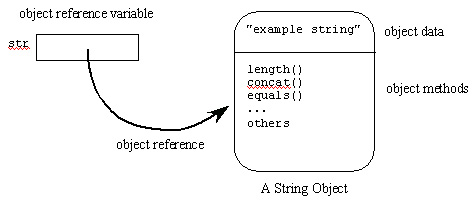The data section of the object contains the characters. The methods section of the object contains many methods.

4. An object reference is information on how to find a particular object. The object is a chunk of main memory; a reference to the object is a way to get to that chunk of memory. The variable str does not actually contain the object, but contains information about where the object is.

5. Objects are created while a program is running. Each object has a unique object reference, which is used to find it. When an object reference is assigned to a variable, then that variable says how to find that object.

C. Variable Versus Object Reference Assignment

1. Notice that there is a difference between the two statements:

`primitiveValue = 18234;`

and

`str = new String("example string");`

In the first statement, `primitiveValue` is a primitive type, so the assignment statement puts the data directly into it. In the second statement, `str` is an object reference variable (the only other possibility) so a reference to the object is put into that variable.

2. A variable will never contain an object. There are only primitive variables and object reference variables, and each contains a specific kind of information:

 Kind of Variable Information it Contains When on the left of "=" primitive variable Contains actual data Previous data is replaced with new data. reference variable Contains information on how to find an object. Old reference is replaced with a new reference

3. The two types of variable are distinguished by how they are declared. Unless it was declared to be of a primitive type, it is an object reference variable. A variable will not change its declared type.

D. Object References

1. It is possible to reassign an object reference to a new value. For example:

```class OneStringReference
{
public static void main (String[] args)
{
String str;

str = new String("first string");
System.out.println(str);

str = new String("second string");
System.out.println(str);
}
}

Run Output:

first string
second string```

2. Notice that:

a. Each time the new operator is used, a new object is created.
b. Each time an object is created, there is a reference to it.
c. This reference is saved in a variable.
d. Later on, the reference in the variable is used to find the object.
e. If another reference is saved in the variable, it replaces the previous reference.
f. If no variables hold a reference to an object, there is no way to find it, and it becomes "garbage."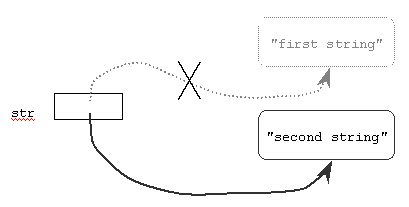3. The word "garbage" is the correct term from computer science to use for objects that have no references. This is a commonly occurring situation, not usually a mistake. As a program executes, a part of the Java system called the "garbage collector" reclaims the lost objects (the "garbage") so that their memory can be used again.

4. Multiple objects of the same class can be maintained by creating unique reference variables for each object.

```class TwoStringReferences
{
public static void main (String[] args)
{
String strA;  // reference to the first object
String strB;  // reference to the second object

// create the first object and save its reference
strA = new String("first string");

// print data referenced by the first object.
System.out.println(strA);

// create the second object and save its reference
strB = new String("second string");

// print data referenced by the first object.
System.out.println(strB);

// print data referenced by the second object.
System.out.println(strA);
}
}

Run Output:

first string
second string
first string```

This program has two reference variables,` strA` and `strB`. It creates two objects and places each reference in one of the variables. Since each object has its own reference variable, no reference is lost, and no object becomes garbage (until the program has finished running.)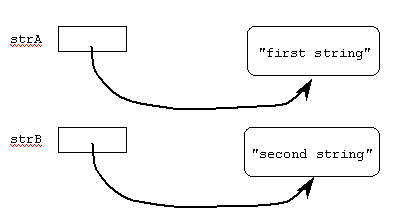5. Different reference variables that refer to the same object are called aliases. In effect, there are two names for the same object. For example:

```class Alias
{
public static void main (String[] args)
{
String strA;  // reference to the object
String strB;  // another reference to the object

// Create the only object and save its
//   reference in strA
strA = new String("only one string");
System.out.println(strA);

strB = strA;  // copy the reference to strB.
System.out.println(strB);
}
}

Run Output:

only one string
only one string```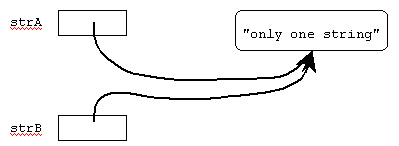When this program runs, only one object is created (by new). Information about how to find the object is put into strA. The assignment operator in the statement

`strB = strA; // copy the reference to strB.`

copies the information that is in strA to strB. It does not make a copy of the object.

E. The == operator with Variables and Object References.

1. The == operator is used to look at the contents of two reference variables. If the contents of both reference variables is the same, then the result is` true`. Otherwise the result is``` false```.

```class EqualsEquals
{
public static void main (String[] args)
{
String strA;  // reference to the first object
String strB;  // reference to the second object

// create the first object and save its reference
strA = new String("same characters");
System.out.println(strA);

// create the second object and save its reference
strB = new String("same characters");
System.out.println(strB);

if (strA == strB)
System.out.println("This will not print.");
}
}

Run Output:

same characters
same characters```

2. In this program, there are two completely separate objects, each of which happens to contain character data equivalent to that in the other. Each object consists of a section of main memory completely separate from the memory that makes up the other object. The variable strA contains information on how to find the first object, and the variable strB contains information on how to find the second object

Since the information in strA is different from the information in strB, (strA == strB) is false, for the first comparison. Since there are two objects, made out of two separate sections of main memory, the reference stored in strA is different from the reference in strB. It doesn't matter that the data inside the objects looks the same.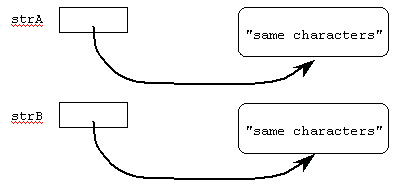3. The == operator does not look at objects. It only looks at references (information about where an object is located.)

4. For primitive types, the == operator looks only at the variables. For example:

```int x = 32;
int y = 48;

if (x == y)                                // false, 32 != 48
System.out.println("They are equal");

X = y;

if (x == y)                                // true, 48 == 48
System.out.println("Now they are equal");

Run Output:

Now they are equal
```

In this code, only the contents of the variables x and y are examined. But with primitive types, the contents of a variable is the data, so with primitive types == looks at data.

5. With primitive and reference types, == looks at the contents of the variables. However, with reference types, the variables contain object references and with primitive types, the variables contain the actual data values.

F. The equals() Method.

1. You have previously seen the equals(String) method of class String. The equals(String) method of class String tests if two Strings contain the same characters.

2. The equals(String) method does look at the contents of objects. It detects "equivalence." The == operator detects "identity". For example,

```String strA;  // first object
String strB;  // second object

strA = new String("different object, same characters");
strB = new String("different object, same characters ");

if (strA == strB)
System.out.println("This will NOT print");

if (strA.equals(strB))
System.out.println("This WILL print");

Run Output:

This WILL print
```

3. In this example, there are two objects. Since each object has its own identity, == reports false. Each object contains equivalent data so equals() reports true.

G. The null Value

1. In most programs, objects are created and objects are destroyed, depending on the data and on what is being computed. A reference variable sometimes does and sometimes does not refer to an object. You need a way to say that a variable does not now refer to an object. You do this by assigning null to the variable.

2. The value null is a special value that means "no object." A reference variable is set to null when it is not referring to any object.

```class nullDemo
{
public static void main (String[] arg)
{
String a = "abracadabra"; // 1. an object is created;
//    variable a refers to it
String b = null;          // 2. variable b refers to no
//    object.
String c = "";            // 3. an object is created
//    (containing no characters)
//     variable c refers to it
if (a != null)            // 4. is true, so the
System.out.println(a); // 5. the println(a) executes.

if (b != null)            // 6. is false, so the
System.out.println(b); // 7. println(b) is skipped.

if (c != null)            // 8. is true, so the
System.out.println(c); // 9. println(c) executes (but
//    it has no characters to
//    print).
}
}

Run Output: